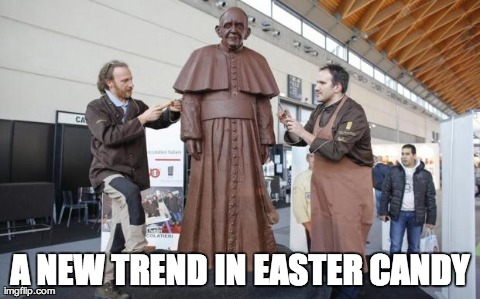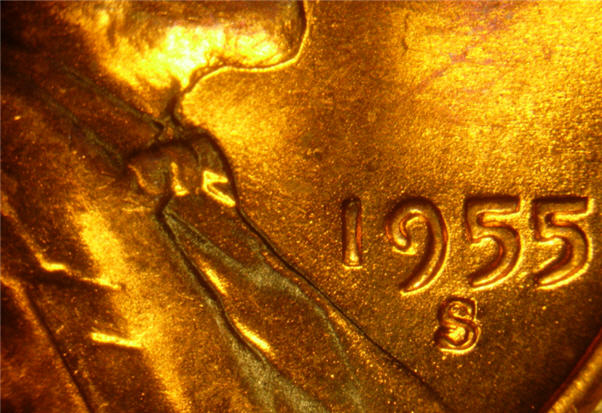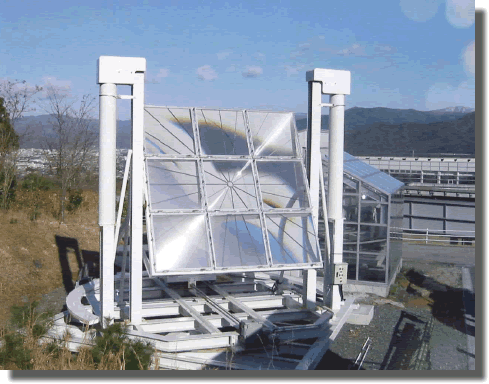Classical Mechanics

# Phase transitions: Level 2-4 ChallengesA cup of warm water is suspended in a large pot of water held at a steady boil at atmospheric pressure. Will the water in the cup ever boil?

Assume that the pot never runs out of water and that the environment remains unchanged.If the chocolate pope is not to be melt on a hot sunny day, what should he be made of?You've found a stockpile of 1955 United States pennies, which are now worth approximately twice as much as raw metal compared to their value as currency. A Fresnel lens can be used to concentrate incoming light onto a focal point.For how many seconds would you need to focus the Fresnel lens on a penny in order to melt the entire coin?

Details and assumptions:

• A penny weighs $2.5 \text{ g}$ and is made of $100$% $\text{Cu}.$
• The penny starts out at $25^\circ\text{C}$ and melts at $1085^\circ\text{C}.$
• $\text{Cu}$'s heat of melting is $176 \text{ kJ}/\text{kg}$ and its specific heat is $0.386\text{ kJ}/\text{kg}\cdot\text{K}.$
• The area of the lens is $0.2 \text{ m}^2,$ the power of the sun is $1370\text{ W/m}^2,$ and $100$% of the energy goes into heating the coin.Suppose you have a glass of water that contains an ice cube. What happens to the water level in the glass when the ice melts?Suppose that the free energy of a system is described by $\mathcal{F}=(T-T_c)m^2+\frac14 m^4$ where $T$ is the temperature, and $m$ represents some property of the system, such as its overall magnetization. As $T$ rises and falls, but does not cross $T_c$, the allowed physical value(s) of $m$, $m^*$ change somewhat (see Assumptions).

When $\displaystyle T$ crosses $T_c$, however, there is a qualitative shift in the allowed values of $m^*$, and the system undergoes a phase transition.

Suppose we're working with the system at a temperature $\displaystyle T$ below $T_c$ such that $T-T_c=-10$, find $\lvert m^*\rvert$.

Assumptions

• The system always resides in states $m^*$ which minimize its free energy. These are the "physical" states of the system.
×